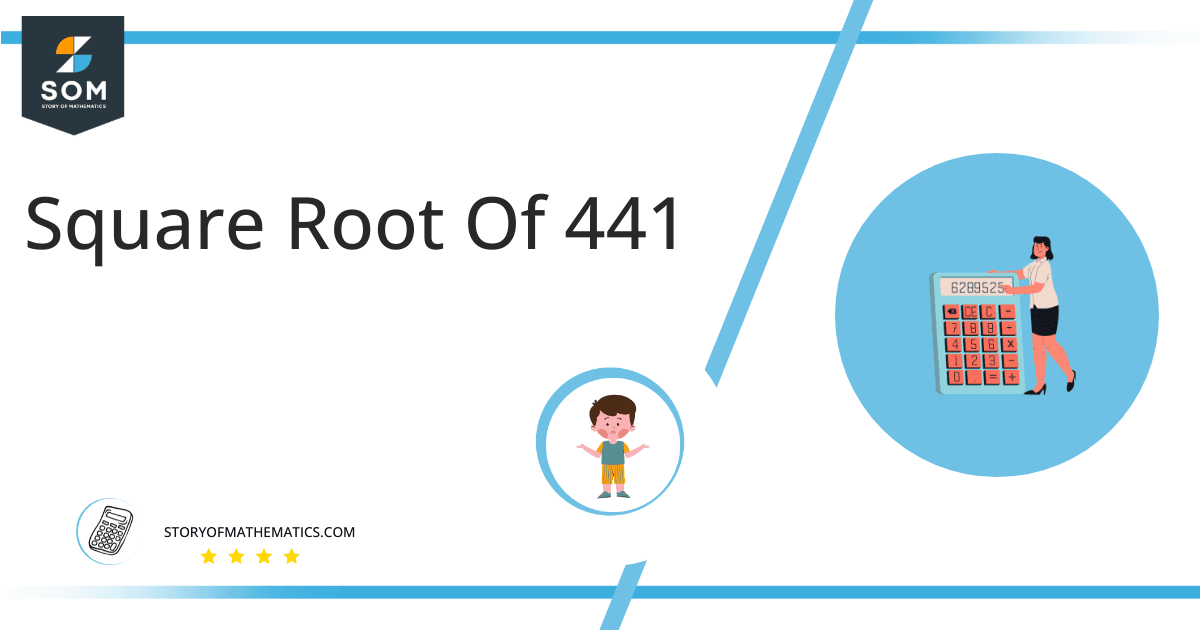# Square Root of 441 + Solution With Free Steps441 is a perfect square and its square root is equal to 21. The square root of 441 will be expressed as √441 = 21, whereis the square root symbol. The square root of a number is that number raised to the power 1/2. For example, the square root of  169 will be 13 as the square of 13 is canceled by the square root.

In this article, we will analyze and find the square root of 441 using various mathematical techniques, such as the approximation method and the long division method.

## What Is the Square Root Of 441?

The square root of the number 441 is 21.

The square root can be defined as the quantity that can be doubled to produce the square of that similar quantity. In simple words, it can be explained as:

√441 = √(21 x 21)

√441 = √(21)$^2$

√441 = ±21

The square can be canceled with the square root as it is equivalent to 1/2; therefore, obtaining 21. Hence 21 is 441’s square root. The square root generates both positive and negative integers.

## How To Calculate the Square Root of 441?

You can calculate the square root of 441 using any of two vastly used techniques in mathematics; one is the Approximation technique, and the other is the Long Division method.

The symbol √ is interpreted as 441 raised to the power 1/2. So any number, when multiplied by itself, produces its square, and when the square root of any squared number is taken, it produces the actual number.

Let us discuss each of them to understand the concepts better.

### Square Root by Long Division Method

The process of long division is one of the most common methods used to find the square roots of a given number. It is easy to comprehend and provides more reliable and accurate answers. The long division method reduces a multi-digit number to its equal parts.

Learning how to find the square root of a number is easy with the long division method. All you need are five primary operations- divide, multiply, subtract, bring down or raise, then repeat.

Following are the simple steps that must be followed to find the square root of 441 using the long division method:

### Step 1

First, write the given number 441 in the division symbol, as shown in figure 1.

### Step 2

Starting from the right side of the number, divide the number 441 into pairs such as 41 and 4 .

### Step 3

Now divide the digit 4 by a number, giving a number either 4 or less than 4. Therefore, in this case, the remainder is zero, whereas the quotient is 2.

### Step 4

After this, bring down the next pair 41. Now the dividend is 41. To find the next divisor, we need to double our quotient obtained before. Doubling 2 gives 4; hence consider it as the next divisor.

### Step 5

Now pair 4 with another number to make a new divisor that results in $\leq$ 41 when multiplied with the divisor. If the number is not a perfect square, add pair of zeros to the right of the number before starting division.

### Step 6

Adding 1 to the divisor and multiplying 41 with 1 results in 41=41. The remainder obtained is 0.

### Step 7

Keep on repeating the same steps till the zero remainder is obtained or if the division process continues infinitely, solve to two decimal places.

### Step 8

The resulting quotient 21 is the square root of 441. Figure 1 given below shows the long division process in detail:### Important points

• The number 441 is a perfect square.
• The number 441 is a rational number.
• The number 441 can be split into its prime factorization.

## Is Square Root of 441 a Perfect Square?

The number 441 is a perfect square. A number is a perfect square if it splits into two equal parts or identical whole numbers. If a number is a perfect square, it is also rational.

A number expressed in p/q form is called a rational number. All the natural numbers are rational. A square root of a perfect square is a whole number; therefore, a perfect square is a rational number.

A number that is not a perfect square is irrational as it is a decimal number. As far as 441 is concerned, it is a perfect square. It can be proved as below:

Factorization of 441 results in 21 x 21 which can also be expressed as 21$^2$.

Taking the square root of the above expression gives:

= √(21$^2$)

= (21$^2$)$^{1/2}$

= 21

This shows that 441 is a perfect square and a rational number.

Therefore the above discussion proves that the square root of 441 is equivalent to 21.Images/mathematical drawings are created with GeoGebra.